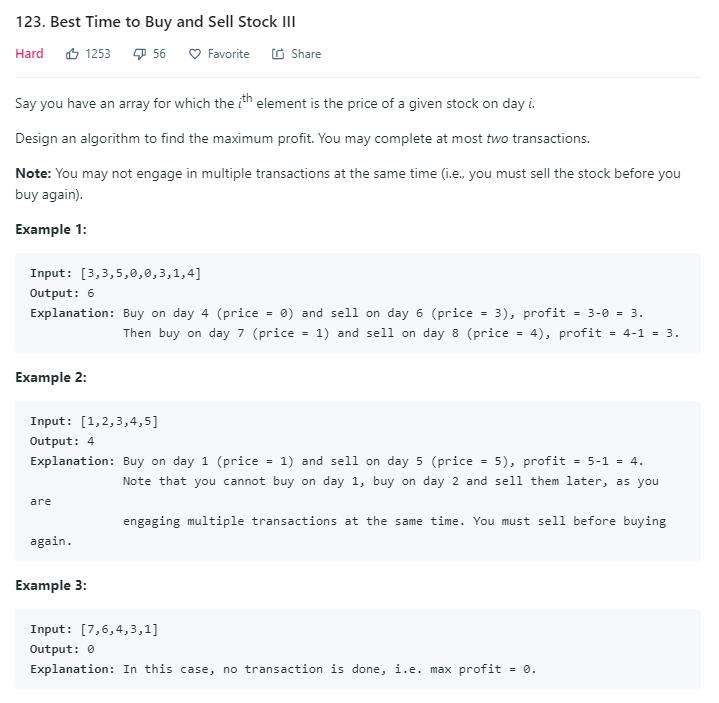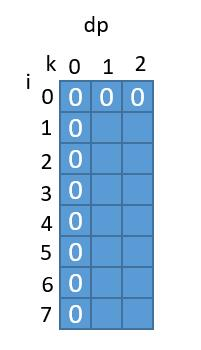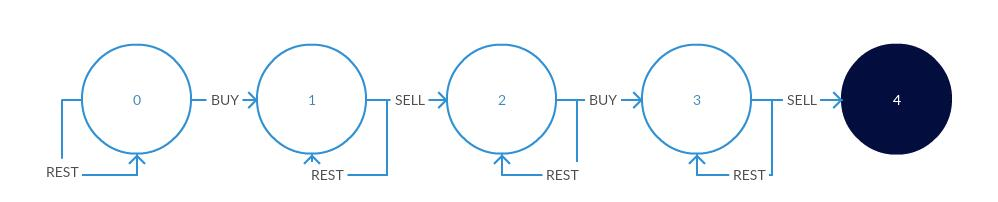# 题目描述（困难难度）# 解法一

1 5 2 8 3 10


dp[i][k] 表示前i天最多交易k次的最高收益，那么 dp[i][k] 怎么通过之前的解求出来呢？

dp[i][k] = dp[i-1][k]

...

dp[i][k] = Max(dp[i-1][k],(prices[i] - prices + dp[k-1]),(prices[i] - prices + dp[k-1])...(prices[i] - prices[i] + dp[i][k-1]))

dp[i][k] = Max(dp[i-1][k],prices[i] - prices[j] + dp[j][k-1])j0 - i

prices[i] - prices[j] + dp[j][k-1] 也可以看做， prices[i] - (prices[j] - dp[j][k-1]) ，为了求这个表达式的最大值，我们可以找prices[j] - dp[j][k-1]的最小值。

public int maxProfit(int[] prices) {
if (prices.length == 0) {
return 0;
}
int K = 2;
int[][] dp = new int[prices.length][K + 1];
for (int k = 1; k <= K; k++) {
for (int i = 1; i < prices.length; i++) {
int min = Integer.MAX_VALUE;
}
//比较不操作和选择一天买入的哪个值更大
dp[i][k] = Math.max(dp[i - 1][k], prices[i] - min);
}
}
return dp[prices.length - 1][K];
}


public int maxProfit(int[] prices) {
if (prices.length == 0) {
return 0;
}
int K = 2;
int[][] dp = new int[prices.length][K + 1];
for (int k = 1; k <= K; k++) {
int min = prices;
for (int i = 1; i < prices.length; i++) {
min = Math.min(prices[i] - dp[i][k - 1], min);
//比较不操作和选择一天买入的哪个值更大
dp[i][k] = Math.max(dp[i - 1][k], prices[i] - min);
}
}
return dp[prices.length - 1][K];
}public int maxProfit(int[] prices) {
if (prices.length == 0) {
return 0;
}
int K = 2;
int[][] dp = new int[prices.length][K + 1];
int min[] = new int[K + 1];
for (int i = 1; i <= K; i++) {
min[i] = prices;
}
for (int i = 1; i < prices.length; i++) {
for (int k = 1; k <= K; k++) {
min[k] = Math.min(prices[i] - dp[i][k - 1], min[k]);
dp[i][k] = Math.max(dp[i - 1][k], prices[i] - min[k]);
}
}
return dp[prices.length - 1][K];
}public int maxProfit(int[] prices) {
if (prices.length == 0) {
return 0;
}
int K = 2;
int[] dp = new int[K + 1];
int min[] = new int[K + 1];
for (int i = 1; i <= K; i++) {
min[i] = prices;
}
for (int i = 1; i < prices.length; i++) {
for (int k = 1; k <= K; k++) {
min[k] = Math.min(prices[i] - dp[k - 1], min[k]);
dp[k] = Math.max(dp[k], prices[i] - min[k]);
}
}
return dp[K];
}


public int maxProfit(int[] prices) {
if (prices.length == 0) {
return 0;
}
int dp1 = 0;
int dp2 = 0;
int min1 = prices;
int min2 = prices;
for (int i = 1; i < prices.length; i++) {
min1 = Math.min(prices[i] - 0, min1);
dp1 = Math.max(dp1, prices[i] - min1);

min2 = Math.min(prices[i] - dp1, min2);
dp2 = Math.max(dp2, prices[i] - min2);
}
return dp2;
}


# 解法二s0代表初始状态，初始时钱是 0s1代表第一次买入后当前的钱，s2代表第一次卖出后当前的前，s3代表第二次买入后当前的钱，s4代表第二次卖出后当前的钱。

int maxProfit(vector<int>& prices) {
if(prices.empty()) return 0;
//进行初始化，第一天 s1 将股票买入，其他状态全部初始化为最小值
int s1=-prices,s2=INT_MIN,s3=INT_MIN,s4=INT_MIN;

for(int i=1;i<prices.size();++i) {
s1 = max(s1, -prices[i]); //买入价格更低的股
s2 = max(s2, s1+prices[i]); //卖出当前股，或者不操作
s3 = max(s3, s2-prices[i]); //第二次买入，或者不操作
s4 = max(s4, s3+prices[i]); //第二次卖出，或者不操作
}
return max(0,s4);
}